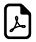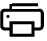Four Times Tables - Doubling-up Trick4 Times Multiplication Table Tricks

An online four times table tricks.Easy way to learn the 4 times table.Four Times Table Easy Learningcan write to
can write to
can write to
can write to
can write to
can write to
can write to
can write to
can write to
can write to
can write to

How 4 times table works?

When you multiply 4 with anything, you have to use the doubling-up trick (that's the one you used for the two times table) twice. Here's an example: 2 x 4 is the same as 2 + 2 = 4 and then 4 + 4 = 8. So 2 x 4 = 8. Here's another double, double example: 5 x 4 is the same as 5 + 5 = 10, so then 10 + 10 = 20. So the answer is 5 x 4 = 20.

Think 4 times.Think Double, Double Trouble !!!

What math times time do you want to generate ?Times Table Tricks 2 to 11►

2 Times Table Trick

4 Times Table Trick

5 Times Table Trick

6 & 7 Times Table Trick

8 Times Table TrickTop Calculators ►

Tax Calculator, Calories Burned Calculator, PERT, SD Calculator, Dog Age Calculator, Children Equal Playing Time Calculator